# Fraction calculator

The calculator performs basic and advanced operations with fractions, expressions with fractions combined with integers, decimals, and mixed numbers. It also shows detailed step-by-step information about the fraction calculation procedure. Solve problems with two, three, or more fractions and numbers in one expression.

## Result:

### 21/2 - 2/3 = 11/6 = 1 5/6 ≅ 1.8333333

Spelled result in words is eleven sixths (or one and five sixths).

### How do you solve fractions step by step?

1. Conversion a mixed number 2 1/2 to a improper fraction: 2 1/2 = 2 1/2 = 2 · 2 + 1/2 = 4 + 1/2 = 5/2

To find a new numerator:
a) Multiply the whole number 2 by the denominator 2. Whole number 2 equally 2 * 2/2 = 4/2
b) Add the answer from previous step 4 to the numerator 1. New numerator is 4 + 1 = 5
c) Write a previous answer (new numerator 5) over the denominator 2.

Two and one half is five halfs
2. Subtract: 5/2 - 2/3 = 5 · 3/2 · 3 - 2 · 2/3 · 2 = 15/6 - 4/6 = 15 - 4/6 = 11/6
For adding, subtracting, and comparing fractions, it is suitable to adjust both fractions to a common (equal, identical) denominator. The common denominator you can calculate as the least common multiple of both denominators - LCM(2, 3) = 6. In practice, it is enough to find the common denominator (not necessarily the lowest) by multiplying the denominators: 2 × 3 = 6. In the following intermediate step, the fraction result cannot be further simplified by canceling.
In other words - five halfs minus two thirds = eleven sixths.

#### Rules for expressions with fractions:

Fractions - simply use a forward slash between the numerator and denominator, i.e., for five-hundredths, enter 5/100. If you are using mixed numbers, be sure to leave a single space between the whole and fraction part.
The slash separates the numerator (number above a fraction line) and denominator (number below).

Mixed numerals (mixed fractions or mixed numbers) write as integer separated by one space and fraction i.e., 1 2/3 (having the same sign). An example of a negative mixed fraction: -5 1/2.
Because slash is both signs for fraction line and division, we recommended use colon (:) as the operator of division fractions i.e., 1/2 : 3.

Decimals (decimal numbers) enter with a decimal point . and they are automatically converted to fractions - i.e. 1.45.

The colon : and slash / is the symbol of division. Can be used to divide mixed numbers 1 2/3 : 4 3/8 or can be used for write complex fractions i.e. 1/2 : 1/3.
An asterisk * or × is the symbol for multiplication.
Plus + is addition, minus sign - is subtraction and ()[] is mathematical parentheses.
The exponentiation/power symbol is ^ - for example: (7/8-4/5)^2 = (7/8-4/5)2

#### Examples:

subtracting fractions: 2/3 - 1/2
multiplying fractions: 7/8 * 3/9
dividing Fractions: 1/2 : 3/4
exponentiation of fraction: 3/5^3
fractional exponents: 16 ^ 1/2
adding fractions and mixed numbers: 8/5 + 6 2/7
dividing integer and fraction: 5 ÷ 1/2
complex fractions: 5/8 : 2 2/3
decimal to fraction: 0.625
Fraction to Decimal: 1/4
Fraction to Percent: 1/8 %
comparing fractions: 1/4 2/3
multiplying a fraction by a whole number: 6 * 3/4
square root of a fraction: sqrt(1/16)
reducing or simplifying the fraction (simplification) - dividing the numerator and denominator of a fraction by the same non-zero number - equivalent fraction: 4/22
expression with brackets: 1/3 * (1/2 - 3 3/8)
compound fraction: 3/4 of 5/7
fractions multiple: 2/3 of 3/5
divide to find the quotient: 3/5 ÷ 2/3

The calculator follows well-known rules for order of operations. The most common mnemonics for remembering this order of operations are:
PEMDAS - Parentheses, Exponents, Multiplication, Division, Addition, Subtraction.
BEDMAS - Brackets, Exponents, Division, Multiplication, Addition, Subtraction
BODMAS - Brackets, Of or Order, Division, Multiplication, Addition, Subtraction.
GEMDAS - Grouping Symbols - brackets (){}, Exponents, Multiplication, Division, Addition, Subtraction.
Be careful, always do multiplication and division before addition and subtraction. Some operators (+ and -) and (* and /) has the same priority and then must evaluate from left to right.

## Fractions in word problems:

• Mountain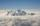Mountain has an elevation of 7450 meters and in the morning is the middle portion thereof in the clouds. How many meters of height is in the sky if below the clouds are 2,000 meters, and above clouds are two-fifths of the mountain's elevation?
• Ali bought 2Ali bought 5/6 litre of milk. He drank 1/2 litre and his brother drank 1/6 litre. How much litre of milk left?
• Mixed numbers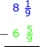Five and two-thirds minus 2 and one-half equals what number? A three and one-sixth B three and two-thirds C three and one-half D three and five-sixths
• 5 2/55 2/5 hours a week  mathematics,  3 3/4 hours a week   Natural sciences, 4 3/8 hours a week  Technology . how many hours does  he spend on social sciences if he spend 17 1/2 hours a week for the four subject?
• Translate 2Translate the given phrases to mathematical phrases. Thrice the sum of three fifths and two thirds less one half is what number?
• SavingsEva borrowed 1/3 of her savings to her brother, 1/2 of savings spent in the store and 7 euros left. How much did she save?
• Jose studiedJose studied for 4 and 1/2 hours on Saturday and another 6 and 1/4 hours on Sunday. How many subjects did he study if he has alloted 1 and 1/2 hours per subject on Saturday and 1 and 1/4 hours per subject on Sunday?
• Mother 7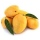Mother bought 18 fruits. 1/3 were pineapple and the rest  were mangoes . how many were mangoes
• Product and sumWhat is the product of two fourths  and the sum of three halves and four?
• PoundsThree pounds subtract 1/3 of a pound.
• From a 2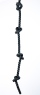From a rope that is 11 m long, two pieces of lengths 13/5 m and 33/10 m are cut off. What is the length of the remaining rope?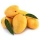A basket contains three types of fruits weighing 87/4 kg in all. If 23/4 kilograms of these are oranges, 48/7 kg are mangoes, and the rest are apples. What is the weight of the apples in the basket?Half of the square we cut off, then half of the rest, etc. Five cuts we made in this way. What part of the content of the original square is the content of the cut part?